## Differentiation Review Page

Although you will likely not use the definition to compute derivatives in later calculus courses, you should nevertheless know the definition of the derivative. The derivative of f with respect to x is given by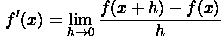when this limit exists.

If f(x) and g(x) are differentiable functions, then the derivative of the product fg with respect to x is given by

(fg)' = f 'g + f g'

This can be fairly easily derived from the definition.

Example

To calculate the derivative of h(x) = x2ex we must use the product rule: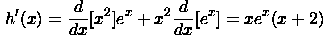If f(x) and g(x) are differentiable functions and g(x) is not equal to zero, then the derivative of the quotient f/g is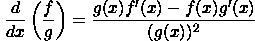which can be remembered with the saying "bottom-derivative-top minus top-derivative-bottom over bottom squared". Although a little harder to do, this result can be derived from the definition. Alternatively, the chain rule can be used to more easily establish this result.

Example

We can use the quotient rule to differentiate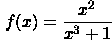Proceeding we have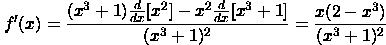If f(x) and g(x) are differentiable functions, then the derivative of the composition of g with f is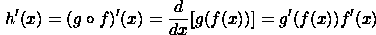where the notation g'(f(x)) means the function g'(x) evaluated at f(x). Once again, this result can be established from the definition.

Example

Since the function h(x) = (2x4 + 3x)8 = g(f(x)) is really the composition of the function g(x) = x8 with f(x) = 2x4 + 3x, the derivative of h is

h'(x) = (g o f)'(x) = g'(f(x))f '(x) = 8(2x4 + 3x)7(8x3 + 3)

Example

Differentiate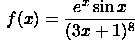We must use all three of the rules we have established (and in the proper order no doubt). The trick is to work from the outside in. The general structure of the function a fraction; thus, we must use the quotient rule: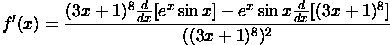But, we notice that in order to carry out the differentiation of the top and bottom terms in the numerator we must use the chain and product rules, respectively. Therefore,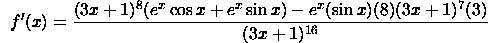which upon simplification becomes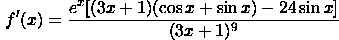Often when carrying out partial differentiation we must use the product, quotient, and chain rules. Several examples follow.

Example

Differentiate f(x,t) = cos(x t2) with respect to t. We must use the chain rule with x held constant. Thus,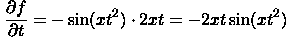Example

Differentiate the following function with respect to y: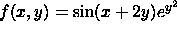To do this, we of course view x as a constant. We see that the product rule must be used since we have a product of two functions involving y.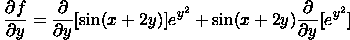But, in taking the derivative of each of the functions sin(x + y) and e^(y2), we must use the chain rule: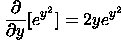Thus,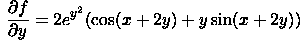Example

To differentiate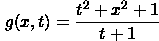with respect to x, it appears we must use the quotient rule. This is actually not the case. Since t is held constant, the denominator is a constant; the only x term appears in the numerator. Thus, the derivative is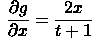Written by Kevin Thompson